< 2015年2月 >
25262728293031
1234567
891011121314
15161718192021
22232425262728
1234567

# 我收藏的一些文章!

•

## 疫苗：Java HashMap的死循环

2013年5月10日发表评论3 人阅读在淘宝内网里看到同事发了贴说了一个CPU被100%的线上故障，并且这个事发生了很多次，原因是在Java语言在并发情况下使用HashMap造成Race Condition，从而导致死循环。这个事情我4、5年前也经历过，本来觉得没什么好写的，因为Java的HashMap是非线程安全的，所以在并发下必然出现问题。但是，我发现近几年，很多人都经历过这个事（在网上查“HashMap Infinite Loop”可以看到很多人都在说这个事）所以，觉得这个是个普遍问题，需要写篇疫苗文章说一下这个事，并且给大家看看一个完美的“Race Condition”是怎么形成的。

#### Hash表数据结构

HashMap通常会用一个指针数组（假设为table[]）来做分散所有的key，当一个key被加入时，会通过Hash算法通过key算出这个数组的下标i，然后就把这个<key, value>插到table[i]中，如果有两个不同的key被算在了同一个i，那么就叫冲突，又叫碰撞，这样会在table[i]上形成一个链表。

#### HashMap的rehash源代码

Put一个Key,Value对到Hash表中：

 123456789101112131415161718192021 `public` `V put(K key, V value)``{``    ``......``    ``//算Hash值``    ``int` `hash = hash(key.hashCode());``    ``int` `i = indexFor(hash, table.length);``    ``//如果该key已被插入，则替换掉旧的value （链接操作）``    ``for` `(Entry e = table[i]; e != ``null``; e = e.next) {``        ``Object k;``        ``if` `(e.hash == hash && ((k = e.key) == key || key.equals(k))) {``            ``V oldValue = e.value;``            ``e.value = value;``            ``e.recordAccess(``this``);``            ``return` `oldValue;``        ``}``    ``}``    ``modCount++;``    ``//该key不存在，需要增加一个结点``    ``addEntry(hash, key, value, i);``    ``return` `null``;``}`

 12345678 `void` `addEntry(``int` `hash, K key, V value, ``int` `bucketIndex)``{``    ``Entry e = table[bucketIndex];``    ``table[bucketIndex] = ``new` `Entry(hash, key, value, e);``    ``//查看当前的size是否超过了我们设定的阈值threshold，如果超过，需要resize``    ``if` `(size++ >= threshold)``        ``resize(``2` `* table.length);``}`

 123456789101112 `void` `resize(``int` `newCapacity)``{``    ``Entry[] oldTable = table;``    ``int` `oldCapacity = oldTable.length;``    ``......``    ``//创建一个新的Hash Table``    ``Entry[] newTable = ``new` `Entry[newCapacity];``    ``//将Old Hash Table上的数据迁移到New Hash Table上``    ``transfer(newTable);``    ``table = newTable;``    ``threshold = (``int``)(newCapacity * loadFactor);``}`

 1234567891011121314151617181920 `void` `transfer(Entry[] newTable)``{``    ``Entry[] src = table;``    ``int` `newCapacity = newTable.length;``    ``//下面这段代码的意思是：``    ``//  从OldTable里摘一个元素出来，然后放到NewTable中``    ``for` `(``int` `j = ``0``; j < src.length; j++) {``        ``Entry e = src[j];``        ``if` `(e != ``null``) {``            ``src[j] = ``null``;``            ``do` `{``                ``Entry next = e.next;``                ``int` `i = indexFor(e.hash, newCapacity);``                ``e.next = newTable[i];``                ``newTable[i] = e;``                ``e = next;``            ``} ``while` `(e != ``null``);``        ``}``    ``}``}`

#### 正常的ReHash的过程

• 我假设了我们的hash算法就是简单的用key mod 一下表的大小（也就是数组的长度）。
• 最上面的是old hash 表，其中的Hash表的size=2, 所以key = 3, 7, 5，在mod 2以后都冲突在table这里了。
• 接下来的三个步骤是Hash表 resize成4，然后所有的<key,value> 重新rehash的过程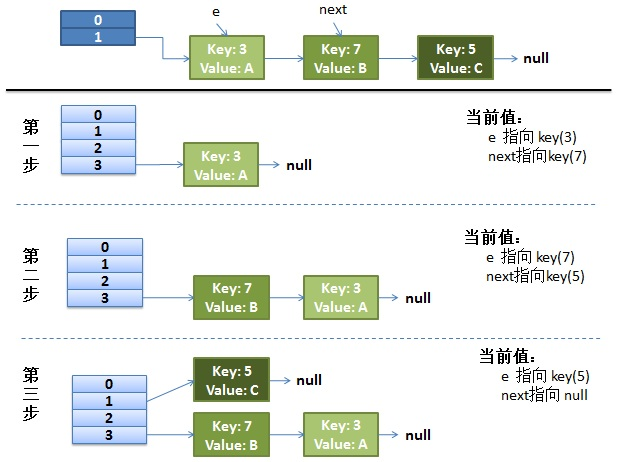#### 并发下的Rehash

1）假设我们有两个线程。我用红色和浅蓝色标注了一下。

 1234567 `do` `{``    ``Entry next = e.next; ``// <--假设线程一执行到这里就被调度挂起了``    ``int` `i = indexFor(e.hash, newCapacity);``    ``e.next = newTable[i];``    ``newTable[i] = e;``    ``e = next;``} ``while` `(e != ``null``);`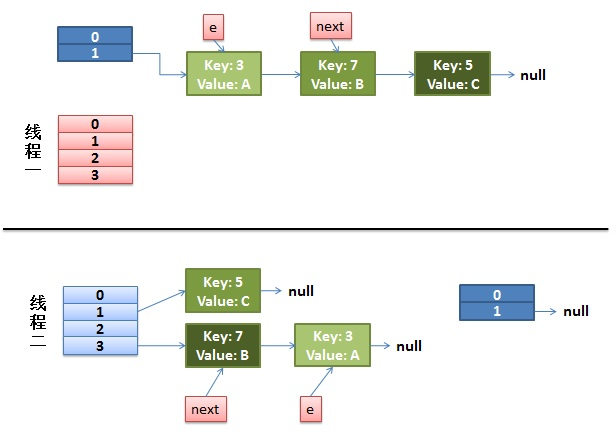2）线程一被调度回来执行。

• 先是执行 newTalbe[i] = e;
• 然后是e = next，导致了e指向了key(7)，
• 而下一次循环的next = e.next导致了next指向了key(3)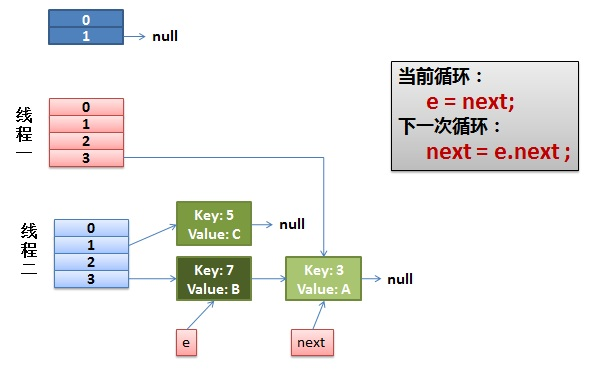3）一切安好。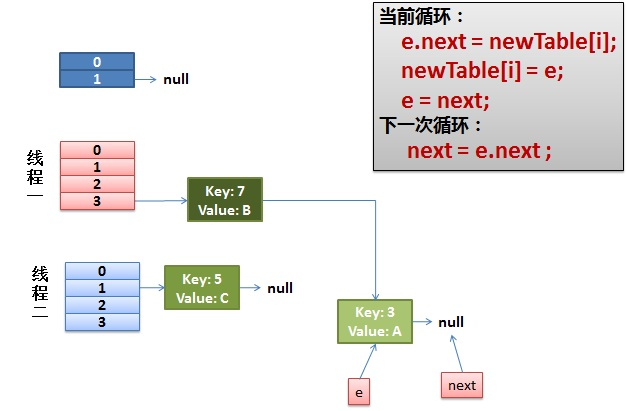4）环形链接出现。

e.next = newTable[i] 导致  key(3).next 指向了 key(7)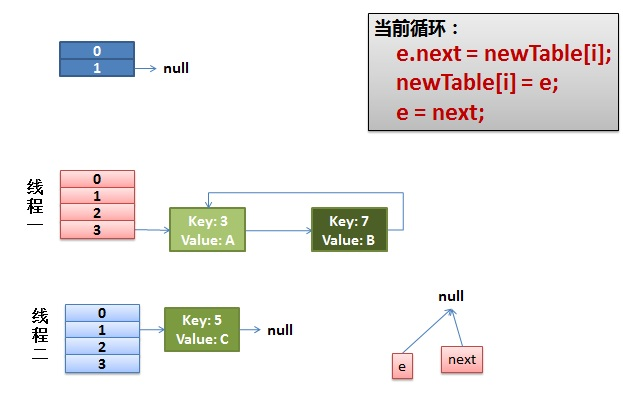#### 其它

http://bugs.sun.com/bugdatabase/view_bug.do?bug_id=6423457

posted on 2015-01-30 17:54 fly 阅读(199) 评论(2)  编辑  收藏 所属分类: java学习FeedBack:
# re: 疫苗：Java HashMap的死循环(转) 2015-01-31 17:52 京山游侠
1.这么好的文章为什么不放到首页？
2.看得出博主和我一样使用的Linux系统，而且主题都和我一样，真高兴能碰到志同道合的朋友。
3.你的图是用什么软件画的？  回复  更多评论

# re: 疫苗：Java HashMap的死循环(转) 2015-02-02 23:12 fly

回复  更多评论

 只有注册用户登录后才能发表评论。 网站导航: 相关文章: